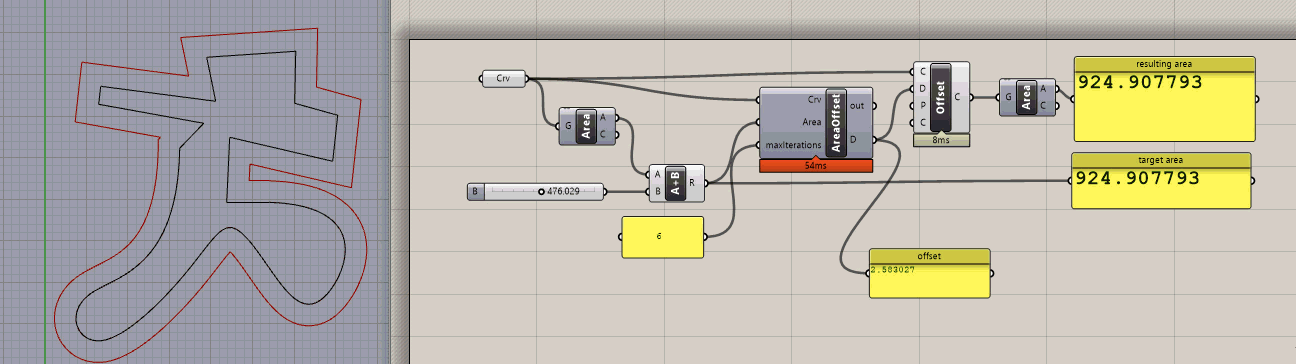# Area and offset distance ratio?

Hi,

How can I find the offset distance required to achieve a certain area.

If I have, as below, a polygon of 190sqm and I need the offset distance d to achieve 120sqm, what is the formula?

For a generic polygon there is not a single solution. Compare an incredibly long thin rectangle with a square. One has the area change nearly entirely due to the long sides, the other due to all sides evenly. Any specific polygon has a solution though.

For small offsets you can approximate the answer by turning the desired area change into a rectangle the length of the polygon perimeter and the width of the offset.

You can use a python or c# component to solve the offset problem to desired accuracy iteratively.

Surely that depends on the triangle? An equilateral triangle will gain less area when offset by some distance than a very thin triangle, because the thin triangle will have relatively large growth in the corners. Or maybe I’m wrong and the differences between the three corners always cancel out…

On the other hand is easy to figure out the 2d scaling factor to go from one area to another.

yes, but in situations like the one below i don’t want to scale…yes, this could be one option but I thought that it could be some mathematical relation…

I could offset many times an pick the curve which has the closest area to what i need…

There isn’t. For square and circles, yes. Easy. For rectangles and triangle, yes. Hard. For arbitrary shapes, no.

ok, thank you, good to know…

Sure, but you posted a triangle, in which case an offset yields a congruent shape.

yeah, i should have posted the U shaped figure…

1 Like

## K=100+70+70-50+60+100=350 dS=So-Sn=6600

4x^2-2Kx+ds=0
4
x^2-2350x+6600=0 => x^2-175*x+1650=0
solving for x (the 2nd root x=155u has not physical meaning):

x=10u

So the offset is 10 u

Similir to this you can derive necessary formulas. Also almost (maybe all?) irregular shapes can be converted to the regular shapes.

3 Likes

Wow, this is impressive @Nosorozec!

@DavidRutten, I think this would be good to have in GH2 as a tool. The main purpose is to control the offset and the area of a building in a plot. Almost all individual buildings per plot have an offset setup by city regulation.

1 Like

@DavidRutten , this is like served on plate!

It works for rectangles:

but when the shape becomes non-rectangular then significant errors creep in. And that’s not even considering cases where the offset begins to self-intersect.

2.5 units squared away from the desired area…

It’s a pretty good way to compute the initial offset attempt, but you just can’t get away from an iterative approach.

@DavidRutten 90% of the buildings on this earth have rectangular footprint or footprint composed of several rectangles.

How does it work for circle and ellipse?

Circle’s way off.

I can add an ‘Offset To Area’ component for curves, but it may yield very unreliable results given how finicky the offset algorithm is sometimes.

Maybe we need another formula from @Mahesh_Ramadas ?

Circles are easy, scaling and offsetting is the same thing, and scaling changes the area in a very straightforward way. The offset of an ellipse is not another ellipse, so I doubt there’s a nice closed form solution for the area of an ellipse offset.

Here’s a fast iterative approach using the same root finding technique I showed here.area_offset.gh (13.2 KB)

5 Likes

You guys are amazingJust put the components into Grasshopper 2 and take my money for Rhino 8